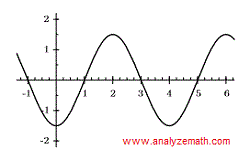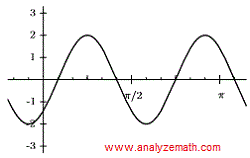# Find Trigonometric Functions Given Their Graphs With Phase Shift (2)

Find the amplitude, period and phase shift of a trigonometric functions given by their graphs. Then find its equation. Questions are presented along with detailed solutions and explanations. Interactive tutorials on Phase Shift and Periodof trigonometric functions may first be used to understand these concepts. Examples on how to find equations of trigonometric graphs with vertical shifts are in part (1)

## Question 1

Find the amplitude, period and phase shift for the curves in 1.a to 1.e, then write the function in the form y = a sin(bx + c).
abcde## Solution

Graph in 1.aFor a function of the form y = a sin(bx + c), the amplitude is given by the maximum value of the function. In graph 1.a, we have:
amplitude: = |a| = 2
We reproduce the graph of 1.a below and note the following:4 small division = π and therefore 1 small division = π/4
One period = 16 small divisions; Hence: 1 period = 16 � π/4 = 4 π
Phase shift: It is the shift between the graphs of y = a sin(bx) and y = a sin(bx + c) and is defined by - c / b.
In the graph of 1.a the phase shift is equal to -π/4 as shown below.(1 small division to the left)We now use the results found above to write an equation of the form y = a sin(bx + c) to the graph in 1.a
|a| = 2, hence a = ~+mn~ 2. let a = 2.
1 period = 4π = 2π / b (assuming b >0). Hence b = 2π / 4π = 1 / 2
Phase shift = -π / 4 = - c / b
Hence c = b � π / 4 = (1 / 2)(π / 4) = π / 8
y = 2 sin (x / 2 + π / 8)

Graph in 1.b
amplitude: = |a| = 1.5
One period = 4
Phase shift = 1 unit to the right = 1
We now use the results found above to write an equation of the form y = a sin(bx + c) to the graph in 1.a
|a| = 1.5, hence a = ~+mn~ 1.5 let a = 1.5
1 period = 4 = 2π / b (assuming b > 0). Hence b = π / 2
Phase shift = 1 = - c / b
Hence c = - b = - π / 2
y = 1.5 sin ( πx / 2 - π / 2)

Graph in 1.c
amplitude: = |a| = 10
1 small division = π / 5 , 1 period = 8 divisions
Hence 1 period = 8 π / 5
Phase shift = 2 divisions = 2π / 5
We now use the results found above to write an equation of the form y = a sin(bx + c) to the graph in 1.a
|a| = 10, hence a = ~+mn~ 10 let a = 10
1 period = 8 π / 5 = 2π / b (assuming b > 0). Hence b = 5 / 4
Phase shift = 2π / 5 = - c / b
Hence c = - 2π b / 5 = - π / 2
y = 10 sin (5 x / 4 - π / 2)

Graph in 1.d
amplitude: = |a| = 3
1 small division = π / 12 , 1 period = 16 divisions
Hence 1 period = 16 � π / 12 = 4 π / 3
Phase shift = 2 divisions to the left = - 2 π / 12 = - π / 6
We now use the results found above to write an equation of the form y = a sin(bx + c) to the graph in 1.a
|a| = 3, hence a = ~+mn~ 3 let a = 3
1 period = 4 π / 3 = 2π / b (assuming b > 0). Hence b = 3 / 2
Phase shift = - π / 6 = - c / b
Hence c = π b / 6 = π / 4
y = 3 sin (3 x / 2 + π / 4)

Graph in 1.e
amplitude: = |a| = 2
1 small division = π / 12 , 1 period = 8 divisions
Hence 1 period = 8 � π / 12 = 2 π / 3
Phase shift = 1 divisions to the right = π / 12
We now use the results found above to write an equation of the form y = a sin(bx + c) to the graph in 1.a
|a| = 2, hence a = ~+mn~ 2 let a = 2
1 period = 2 π / 3 = 2π / b (assuming b > 0). Hence b = 3
Phase shift = π / 12 = - c / b
Hence c = - π b / 12 = - π / 4
y = 2 sin (3 x - π / 4)
As an exercise, graph each of the functions found above and compare the graphs obtained to the graphs given above.

## Question 2

Find the amplitude, period and phase shift for the curves in Problems 2.a to 2.c, then write the function in the form y = a cos(bx + c).
2.a2.b2.c## Solution

Graph in 2.aFor a function of the form y = a cos(bx + c), the amplitude is given by the maximum value of the function. In graph 2.a, we have:
amplitude: = |a| = 4
We reproduce the graph of 1.a below and note the following:
One period = 3 π/ 2
Phase shift: It is the shift between the graphs of y = a cos(bx) and y = a cos(bx + c) and is defined by - c / b.
In the graph of 2.a the phase shift is equal 3 small divisions to the right.
1 small division = π / 8
Phase shift = 3 � π / 3 = 3 π / 8We now use the results found above to write an equation of the form y = a cos(bx + c) to the graph in 2.a
|a| = 4, hence a = ~+mn~ 4; let a = 4
1 period = 3 π/ 2 = 2π / b (assuming b > 0). Hence b = 4 / 3
Phase shift = 3 π / 8 = - c / b
Hence c = - b � 3 π / 8 = - π / 2
y = 4 cos (4 x / 3 - π / 2)

Graph in 2.b
amplitude: = |a| = 3
One period = 1 (length of the x axis of one cycle)
Phase shift = 1 / 2 (half a unit to the right)
We now use the results found above to write an equation of the form y = a cos(bx + c) to the graph in 2.b
|a| = 3, hence a = ~+mn~ 3 let a = 3
1 period = 1 = 2π / b (assuming b > 0). Hence b = 2 π
Phase shift = 1 / 2 = - c / b
Hence c = - b / 2 = - π
y = 3 sin ( 2 π x - π)

Graph in 2.c
amplitude: = |a| = 40
1 small division = (π / 2) / 4 = π / 8
1 period = 8 divisions
Hence 1 period = 8 � π / 8 = π
Phase shift = 3 divisions (to the right) = 3 � π / 8 = 3π / 8
We now use the results found above to write an equation of the form y = a cos(bx + c) to the graph in 2.c
|a| = 40, hence a = ~+mn~ 40 let a = 40
1 period = π = 2π / b (assuming b > 0). Hence b = 2
Phase shift = 3π / 8 = - c / b
Hence c = - 3π b / 8 = - 3π / 4
y = 40 cos (2 x - 3π / 4)
As an exercise, graph each of the functions found above and compare the graphs obtained to the graphs given above.

## Question 3

The graph of the function y = sin(bx + c) has an x-intercept at x = 6π/5 and a maximum at x = 11π/5 as shown below.a) What is the period of the function?
b) Find b and c, and the equation of the graph.

## Solution

a) The absolute value of the difference of the x coordinates of the points (6π/5 , 0) and (11π/5 , 1) gives the quarter of the period. Hence the period P is equal to:
P = 4(11π/5 - 6π/5) = 4π
b)
The period found above is also given by
P = 2π / | b |
Take b positive and solve the equation 2π / b = 4π for b
b = 1 / 2
The phase shift of the graph given above is equal to 6π / 5 and is also given by the formula - c / b. Hence the equation:
- c / b = 6π / 5
Solve for c.
c = - 6π b / 5 = - 3π / 5
y = sin((1/2) x - 3π / 5)